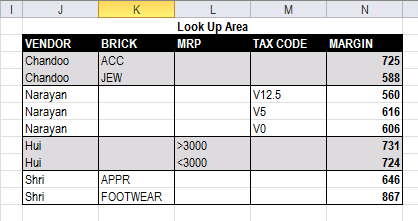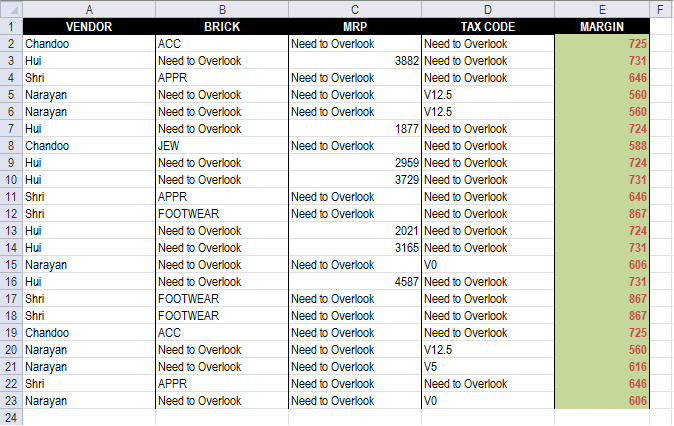Author: Oscar Cronquist Article last updated on March 03, 2023I failed to search for "Ask" Section.. so submitting my query here.. Apologize for hijacking someones post,, and also for cross-post..

https://chandoo.org/forums/topic/lookup-using-multiple-condition

* Each Vendor has only one type of MARGIN decider (MRP or BRICK or TAX Code)
* According to Margin decider, I need to get MARGIN for the VENDOR..

i.e Vendor 1 (Chandoo) 's Margin Decider is BRICK..
If brick is ACC then Margin is 548, if Brick is JEW then Margin is 786.. (Check Table2 for lookupArea and Margin Decider..LookUpArea..* suggestion for changing Design of LookUpArea's is appreciable.
* use of HELPER column is also acceptable..

https://dl.dropbox.com/u/78831150/Excel/MultipleLookup.xlsx

You can get the VBA version for your reference..
https://dl.dropbox.com/u/78831150/Excel/MultipleLookup.xlsm

Regards,
DebArray formula in cell E2:

=INDEX(\$N\$3:\$N\$11, MATCH(1, COUNTIFS(INDEX((B2, C2, D2), , , MATCH(TRUE, B2:D2<>"Need to Overlook", 0)), INDEX((\$K\$3:\$K\$11, \$L\$3:\$L\$11, \$M\$3:\$M\$11), , , MATCH(TRUE, B2:D2<>"Need to Overlook", 0)), A2, \$J\$3:\$J\$11), 0))

### How to enter an array formula

1. Select cell E2
2. Paste array formula to formula bar
3. Press and hold CTRL + SHIFT
4. Press Enter

### How to copy array formula

1. Select cell E2
2. Copy cell E2 (Ctrl + c)
3. Select cell range E3:E23
4. Paste (Ctrl + v)

### Explaining array formula in cell E2

#### Step 1 - Determine which cell range to use

The less than an larger than signs combined checks if a value is not equal to another value, the result si a boolean value TRUE or FALSE.

B2:D2<>"Need to Overlook"

becomes

{"ACC","Need to Overlook","Need to Overlook"}<>"Need to Overlook"

and returns

{TRUE, FALSE, FALSE}

#### Step 2 - Determine which cell range to use

The MATCH function returns the relative position of an item in an array that matches a specified value in a specific order.

Function syntax: MATCH(lookup_value, lookup_array, [match_type])

MATCH(TRUE, B2:D2<>"Need to Overlook", 0)

becomes

MATCH(TRUE,{TRUE, FALSE, FALSE} , 0)

and returns 1.

#### Step 3 - Return cell reference

The INDEX function returns a value or reference from a cell range or array, you specify which value based on a row and column number.

Function syntax: INDEX(array, [row_num], [column_num])

INDEX((B2, C2, D2), , , MATCH(TRUE, B2:D2<>"Need to Overlook", 0))

becomes

INDEX((B2, C2, D2), , , 1)

and returns cell reference B2

#### Step 4 - Find a matching row

The COUNTIFS function calculates the number of cells across multiple ranges that equals all given conditions.

Function syntax: COUNTIFS(criteria_range1, criteria1, [criteria_range2, criteria2]…)

COUNTIFS(INDEX((B2, C2, D2), , , MATCH(TRUE, B2:D2<>"Need to Overlook", 0)), INDEX((\$K\$3:\$K\$11, \$L\$3:\$L\$11, \$M\$3:\$M\$11), , , MATCH(TRUE, B2:D2<>"Need to Overlook", 0)), A2, \$J\$3:\$J\$11)

becomes

COUNTIFS(B2, \$K\$3:\$K\$11, A2, \$J\$3:\$J\$11)

and returns

{1;0;0;0;0;0;0;0;0}.

#### Step 5 - Calculate the relative position of row

The MATCH function returns the relative position of an item in an array that matches a specified value in a specific order.

Function syntax: MATCH(lookup_value, lookup_array, [match_type])

MATCH(1, COUNTIFS(INDEX((B2, C2, D2), , , MATCH(TRUE, B2:D2<>"Need to Overlook", 0)), INDEX((\$K\$3:\$K\$11, \$L\$3:\$L\$11, \$M\$3:\$M\$11), , , MATCH(TRUE, B2:D2<>"Need to Overlook", 0)), A2, \$J\$3:\$J\$11), 0)

becomes

MATCH(1, {1;0;0;0;0;0;0;0;0}, 0)

and returns 1.

#### Step 6 - Return margin value

The INDEX function returns a value or reference from a cell range or array, you specify which value based on a row and column number.

Function syntax: INDEX(array, [row_num], [column_num])

INDEX(\$N\$3:\$N\$11, MATCH(1, COUNTIFS(INDEX((B2, C2, D2), , , MATCH(TRUE, B2:D2<>"Need to Overlook", 0)), INDEX((\$K\$3:\$K\$11, \$L\$3:\$L\$11, \$M\$3:\$M\$11), , , MATCH(TRUE, B2:D2<>"Need to Overlook", 0)), A2, \$J\$3:\$J\$11), 0))

becomes

INDEX(\$N\$3:\$N\$11, 1)

becomes

INDEX({725; 588; 560; 616; 606; 731; 724; 646; 867}, 1)

and returns 725 in cell E2.

### Get the Excel fileMultipleLookup_solution1.xlsx